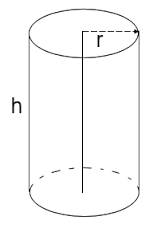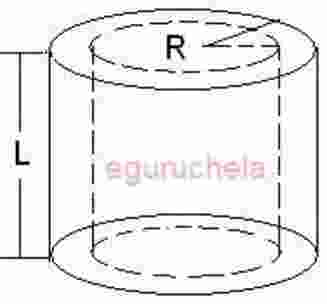# Calculate Volume of Shell of Pipe / Cylinder

Calculates the volume of the shell of a tube or pipe for given radius and length.

 Enter Radius cm Enter Length cm

 Pipe Volume = cm3
Using the following formula:
Volume = Πr2 X Hight or LengthCircular hollow cylinder or pipe, where